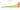# FR

## Rwandan Franc

The national currency of Rwanda is the franc. There are 100 centimes in it.

#### how has the value of the currency changed in the last year?## USD 1 = RWF 1,232.6

How much is10 US dollars worth inRwandan francs?
At the current exchange rate, 10 US dollars is worth 12,325.66 Rwandan francs
How much is50 US dollars worth inRwandan francs?
At the current exchange rate, 50 US dollars is worth 61,628.31 Rwandan francs
How much is100 US dollars worth inRwandan francs?
At the current exchange rate, 100 US dollars is worth 123,256.63 Rwandan francs
How much is500 US dollars worth inRwandan francs?
At the current exchange rate, 500 US dollars is worth 616,283.14 Rwandan francs
How much is2,000 US dollars worth inRwandan francs?
At the current exchange rate, 2,000 US dollars is worth 2,465,132.58 Rwandan francs
How much is10 US dollars worth inRwandan francs?
At the current exchange rate, 10 US dollars is worth 12,325.66 Rwandan francs
How much is50 US dollars worth inRwandan francs?
At the current exchange rate, 50 US dollars is worth 61,628.31 Rwandan francs
How much is100 US dollars worth inRwandan francs?
At the current exchange rate, 100 US dollars is worth 123,256.63 Rwandan francs
How much is500 US dollars worth inRwandan francs?
At the current exchange rate, 500 US dollars is worth 616,283.14 Rwandan francs
How much is2,000 US dollars worth inRwandan francs?
At the current exchange rate, 2,000 US dollars is worth 2,465,132.58 Rwandan francs